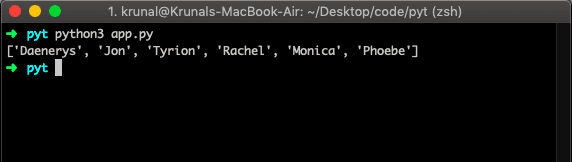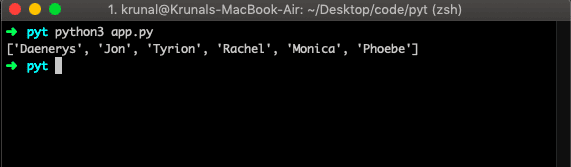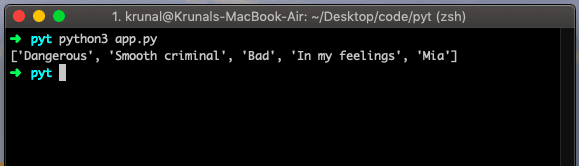# Python list extend: How to Add Elements in the List

Adding elements after creating a list is one of the most frequent operations in Python. The length of the list increases by several elements in its argument.

## Python list extend

The list extend() is a built-in Python method that adds the specified list elements (or any iterable) to the end of the current list. The list.extend() method takes an iterable as argument and does not return any value.

For example, the extend() method appends the contents of seq to the list. It extends the list by adding all list elements (passed as an argument) to the end.

### Syntax

The syntax for the Python list extend() method is the following.

`list.extend(iterable)`

### Parameters

An iterable parameter is required, and it is iterable like set, tuple, list. The list extend() method does not return any value but adds the content to the existing list.

### Example

Let us take an example. My current version of Python is 3.

```# app.py

GoT = ['Daenerys', 'Jon', 'Tyrion']
Friends = ['Rachel', 'Monica', 'Phoebe']
GoT.extend(Friends)
print(GoT)```

Run the file.

`python3 app.py`So, we have added one list to the other, and the output will be six items of the list. It is an entirely different behavior from the Python list append() method.

In that case, we have only four elements, and the 4th one is added as a whole item.

To merge lists in Python, use the list.extend() method.

## How to append elements of Tuple to List.

To append tuple to the list, we can use the list extend() method. First, define a tuple and list and then append tuple to list using extend() method.

Let us extend a tuple to the list.

```# app.py

GoT1_list = ['Daenerys', 'Jon', 'Tyrion']
Friends1_tuple = ('Rachel', 'Monica', 'Phoebe')
GoT1_list.extend(Friends1_tuple)
print(GoT1_list)```

In the above example, we have taken one list and one tuple. Now, we try to add the tuple to a list. The output is the following. It will become a single list.## Appending items of Set To List in

To append a set to a list, we can use the list.extend() method in Python. First, define a list and set and then use the list.extend() method to add a set to the list. See the following code.

```# app.py

MJSongs_list = ['Dangerous', 'Smooth criminal', 'Bad']
DrakeSongs_set = {'In my feelings', 'Mia'}
MJSongs_list.extend(DrakeSongs_set)
print(MJSongs_list)```It becomes one list and includes all the elements in a single list.

The Python native datatypes like tuple and set passed to extend() method is automatically converted to a list. And the items on the list are appended to the end.

## Conclusion

In this example, we have seen how to use a list.extend() method and then use the extend() method to append the set and tuple to the existing list.

Finally, Python List Extend Example is over.

Python list append()

Python list contains

Python list to json

Python list to dictionary

### 2 thoughts on “Python list extend: How to Add Elements in the List”

1.def my_fun(x):
for k in range (len(x)):
print(‘k is ‘,k)
x.extend(x[:k])
print(‘x is’,x)
m = [2,4,3]
my_fun(m)
print(m)
********************************
out put

k is 0
x is [2, 4, 3] Here it did not do any thing
k is 1
x is [2, 4, 3, 2]
k is 2
x is [2, 4, 3, 2, 2, 4] Why 2 is added 2 times?
[2, 4, 3, 2, 2, 4]

2.